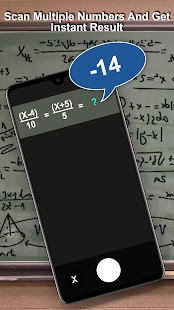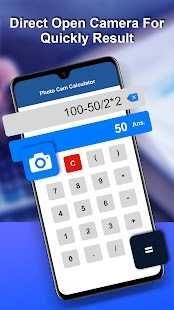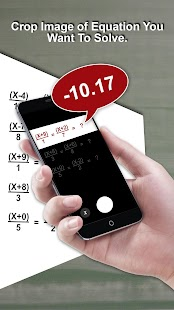Camera Math Calculator is an application that solves your math problems in a seconds. You don’t need to do anything, just capture the picture of the math problem and crop the particular portion of calculation and the application will solve it for you.

Features:
– Calculate math problems with camera.
– Click a photo of any mathematical problem, crop the portion and save it. The app will solve that problem for you.
– The app can solve simple as well as complex math problems.
– Addition, subtraction, multiplication, division, algebra, percentage, emi, interest etc can be solved.
– The app also contains manual calculator, where in you can manually calculate.

What’s New:
No changelog

Screenshots

•••••••••